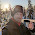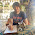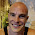## Thursday, February 11, 2016

### Effect stability: (2) Simple mediation designs

In my last post, I described how a significant estimate need not be close to its population value, and how, using a clever method developed by Schönbrodt and Perugini (2013), one can estimate the sample size required to achieve stability for an estimator through simulation.

Schönbrodt's and Perugini's method defines a point of stability (POS), a sample size beyond which one is reasonably confident that an estimate is within a specified range (labeled the corridor of stability, or COS) of its population value.  For more details on how the point of stability is estimated, you can read either my previous post or Schönbrodt's and Perugini's paper.

By adapting Schönbrodt's and Perugini's freely available source code, I found that, in two-group, three-group, and interaction designs, statistical stability generally requires sample sizes around 150-250.  In this post, I will apply this same method to simple mediation designs.

### Simple mediation designs

Simple mediation designs involve three variables: a predictor variable $X$ (which is usually manipulated), an outcome variable $Y$, and a hypothesized mechanism (mediator) for the relationship between $X$ and $Y$, $M$.  Assuming that there is a causal relationship between $X$ and $Y$, $M$ a simple mediation design aims to establish whether $M$ is part of the reason for this causal relationship, as diagrammed below.

In this diagram, $X$ is connected to $Y$ in two ways -- once via path $c$, and a second time through the two paths $a$ and $b$.  The indirect effect of $X$ on $Y$ through $M$ is therefore the product of paths $a$ and $b$ ($ab$), which is an estimator of the population indirect effect $\alpha\beta$.  If path $c$ is non-zero, $X$ may also have a direct effect on $Y$, which is the effect of $X$ on $Y$ that is not mediated through $M$.  The total effect of $X$ on $Y$ is merely the sum of the indirect and direct effects, $ab + c$.

The paths $a$, $b$, and $c$ can be estimated using the following two statistical models:

$$M = aX + e_1$$
$$Y = bM + cX + e_2$$

Here, coefficient $c$ estimates the direct effect of $X$ on $Y$, and the product $ab$ estimates the indirect effect of $X$ on $Y$ through $M$.  Thus, the population indirect effect $\alpha\beta$ of $X$ on $Y$ through $M$ can be estimated using the above two statistical models by taking the product $ab$.

### Effect sizes for the indirect effect

What I have described so far is standard and well-known about simple mediation designs.  What is less obvious is the proper estimator one should use to measure the size of the indirect effect.

One of the more obvious options is to use standardized estimates of $\alpha$ and $\beta$ and simply calculate their product.  Thus, for $\alpha$, one could estimate the correlation between $X$ and $M$, and, for $\beta$, one could estimate the semipartial correlation between $M$ and $Y$ (controlling for $X$).  Their product, which I will call $ab_{\text{standard}}$, yields a standardized estimate of the indirect effect.

Other commonly used alternatives express the magnitude of $ab$ relative to other quantities in a simple mediation model.  The most common approach is to estimate the ratio between the indirect and total effects.  Mathematically, this reduces to estimating the proportion $P_M$ as follows:

$$P_M=\frac{ab}{ab + c}$$

$P_M$ is not a true proportion in that it can take on values that are negative or greater than one, which hinders its interpretability.  However, $P_M$ can be easily estimated using the same two models used to estimate $\alpha\beta$.  $P_M$ also has some intuitive appeal as a comparison between two of the important quantities in mediation analysis, the indirect and total effects.

Overall, there is little consensus about the best way to measure the size of the indirect effect -- in a recent paper, Preacher and Kelley (2011) describe fully 16 options.  As the estimators most commonly used, I will focus on $ab_{\text{standard}}$ and $P_M$.

### Estimating the stability of an indirect effect estimator

To apply Schönbrodt's and Perugini's method to a simple mediation model, I created populations with variables $X$, $M$, and $Y$ in which I had systematically varied $\alpha$ and $\beta$ while keeping the total effect constant to $\rho = .4$.  I then drew 10,000 effect size trajectories, drawing an initial small sample and successively recalculating the target effect size metric as additional cases were added to the sample until the size of the sample reached 1000.

Defining a corridor of stability (COS) and its half-width $w$ was a little tricky.  Because there is no broad consensus as to what constitutes a "small", "medium", or "large" value of the indirect effect, there is therefore little guidance as to what might represent a small, medium, or large deviation of an estimate of the indirect effect from its population value.

I used the following logic to choose values for $w$.  Schönbrodt and Perugini argued that values of .1, .15, and .2 represent small, medium, and large deviations from a population $\rho$.  $ab_{\text{standard}}$ is the product of two correlations; thus, $.1^2 = .01$, $.15^2 = .025$ and $.20^2 = .04$ seem like reasonable choices to represent represent small, medium and large deviations from the population indirect effect.  Similarly, $P_M$ is just $ab_{\text{standard}}$ divided by the total effect, which across my simulations I am setting to .4.  Thus, some reasonable values for the half-width of the COS for $P_M$ are $.01/.4= .025$, $.025/.4= .0625$, and $.04/.4=.1$.

For each of $ab_{\text{standard}}$ and $P_M$, I investigated a case where $X$ is quantitative and a case where $X$ is categorical.  The results for a quantitative and categorical $X$ were relatively similar, so I will only be presenting the results for a quantitative $X$.  You can find both my results for a categorical $X$ and my source code here.

### The stability of $ab_{\text{standard}}$

Below are the points of stability for $ab_{\text{standard}}$, given varying values of $\alpha$, $\beta$, the half-width of the corridor of stability $w$, and the confidence of the point of stability.  For reference, I have also included a column giving the value of $P_M$.

$$\begin{array}{cccc|ccc|ccc|ccc} &&&&&80\%&&&90\% &&&95\%&&\\ \alpha&\beta&\alpha\beta&P_M&w = .01&w = .0225 & w = .04 & w = .01 & w = .0225 & w = .04 & w = .01 & w = .0225 & w = .04\\ \hline 0.1&0.1&0.01&0.025&541&133&57&808&204&83&986&296&112\\ 0.1&0.2&0.02&0.05&992&289&98&1000&434&146&1000&600&197\\ 0.1&0.3&0.03&0.075&1000&572&186&1000&805&272&1000&967&366\\ 0.1&0.4&0.04&0.1&1000&872&313&1000&1000&453&1000&1000&604\\ 0.2&0.1&0.02&0.05&959&260&90&1000&391&137&1000&533&186\\ 0.2&0.2&0.04&0.1&1000&411&125&1000&614&192&1000&820&271\\ 0.2&0.3&0.06&0.15&1000&641&204&1000&906&308&1000&1000&423\\ 0.2&0.4&0.08&0.2&1000&902&320&1000&1000&468&1000&1000&631\\ 0.3&0.1&0.03&0.075&1000&492&160&1000&710&236&1000&896&315\\ 0.3&0.2&0.06&0.15&1000&576&185&1000&827&276&1000&984&368\\ 0.3&0.3&0.09&0.225&1000&751&244&1000&976&359&1000&1000&488\\ 0.3&0.4&0.12&0.3&1000&916&337&1000&1000&489&1000&1000&658\\ 0.4&0.1&0.04&0.1&1000&766&267&1000&968&390&1000&1000&510\\ 0.4&0.2&0.08&0.2&1000&802&283&1000&988&404&1000&1000&521\\ 0.4&0.3&0.12&0.3&1000&847&304&1000&1000&432&1000&1000&563\\ 0.4&0.4&0.16&0.4&1000&896&343&1000&1000&482&1000&1000&626\\ \end{array}$$

According to my simulations, the points of stability for $ab_{\text{standard}}$ are extremely high, and these points of stability increase as the population value of the indirect effect increases.  In fact, in many of the cells of the POS table, the point of stability was beyond the maximum N that I sampled in the bootstrapped trajectories (1000).

To see visually what's going on, I have plotted 100 trajectories with two different values of $\alpha\beta$, .01 and .16, along with a COS half-width of $w=.04$.100 simulated trajectories, $\alpha\beta = .01$100 simulated trajectories, $\alpha\beta = .16$

From these graphs, we can see that the trajectories are much more unstable with a larger population value of $\alpha\beta$.  This makes some intuitive sense because fluctuations in either $a$ or $b$ will ramify into fluctuations of $ab_{\text{standard}}$, and the fluctuations will be especially severe if the population values of either $\alpha$ or $\beta$ are large.  Supporting this interpretation, the sampling distribution of $\alpha\beta$ is known to be non-normal due to skewness and excess kurtosis, and the departure from normality depends in part on the values of $\alpha$ or $\beta$ (MacKinnon, Fritz, Williams, & Lockwood, 2007).

It is also instructive to compare the points of stability for the following values of $\alpha$ and $\beta$: $\alpha=.1; \beta=.4$, $\alpha=.2; \beta=.2$, and $\alpha=.4; \beta=.1$.  In these cases, the magnitude of the indirect effect is equivalent.  Nonetheless, the points of stability are consistently higher when either $\alpha$ or $\beta$ is equal to .4 than when they are both equal to .2.

### The stability of $P_M$

Based on the above discussion, one might expect the stability of $P_M$ to be even worse than the stability of $ab_{\text{standard}}$, because the value of $P_M$ depends on the estimated value of the total effect, which is yet another parameter that can take on an extreme value.  Indeed, the table of the points of stability, below, supports this expectation.

$$\begin{array}{cccc|ccc|ccc|ccc} &&&&&80\%&&&90\% &&&95\%&&\\ a&b&ab&P_M&w = .025&w = .05625 & w = .1 & w = .025 & w = .05625 & w = .1 & w = .025 & w = .05625 & w = .1\\ \hline 0.1&0.1&0.01&0.025&562&146&65&824&223&95&982&314&132\\ 0.1&0.2&0.02&0.05&985&286&103&1000&423&156&1000&577&207\\ 0.1&0.3&0.03&0.075&1000&546&182&1000&753&262&1000&930&349\\ 0.1&0.4&0.04&0.1&1000&808&286&1000&995&414&1000&1000&544\\ 0.2&0.1&0.02&0.05&975&280&100&1000&422&155&1000&565&214\\ 0.2&0.2&0.04&0.1&1000&423&133&1000&628&208&1000&835&291\\ 0.2&0.3&0.06&0.15&1000&630&205&1000&880&299&1000&1000&407\\ 0.2&0.4&0.08&0.2&1000&844&294&1000&1000&432&1000&1000&582\\ 0.3&0.1&0.03&0.075&1000&522&179&1000&745&265&1000&931&350\\ 0.3&0.2&0.06&0.15&1000&647&210&1000&888&315&1000&1000&439\\ 0.3&0.3&0.09&0.225&1000&763&270&1000&981&401&1000&1000&534\\ 0.3&0.4&0.12&0.3&1000&898&328&1000&1000&497&1000&1000&672\\ 0.4&0.1&0.04&0.1&1000&797&290&1000&986&421&1000&1000&547\\ 0.4&0.2&0.08&0.2&1000&854&326&1000&1000&466&1000&1000&619\\ 0.4&0.3&0.12&0.3&1000&905&360&1000&1000&521&1000&1000&677\\ 0.4&0.4&0.16&0.4&1000&957&404&1000&1000&569&1000&1000&764\\ \end{array}$$

If we just focus on the two columns where $w = .05625$ and where $w=.1$ (the two larger values of the half-width of the COS), the points of stability are on average 14.52 cases larger for $P_M$ than they are for $ab_{\text{standard}}$, and the problem is particularly acute as $\alpha\beta$ increases.  You can see this pattern in the two sets of trajectories plotted below.100 simulated trajectories, $P_M = .01$100 simulated trajectories, $P_M = .4$

### Conclusions

There are some obvious limitations to my approach.  First, as I pointed out, I do not have a good basis to say what constitutes a "small" or "large" departure from the population indirect effect, so the values that I used to construct the corridors of stability were somewhat arbitrary and could very well have been too restrictive.  Second, I only investigated one value for the total effect -- a generous $\rho = .4$ -- so I can't say with confidence how stability is affected if one systematically varies this value.

However, based on the simulations that I conducted, I think it's reasonable to conclude that obtaining an accurate estimate of the indirect effect is quite difficult.  Although it is possible to obtain reasonable power to detect a non-zero indirect effect at somewhat smaller sample sizes (Fritz & MacKinnon, 2007), obtaining a good or accurate estimate of the indirect effect probably requires a sample size that is extremely large, perhaps even in excess of 1000.

References

Fritz, M. S., & MacKinnon, D. P. (2007). Required sample size to detect the mediated effect. Psychological Science, 18, 233–239. http://doi.org/10.1111/j.1467-9280.2007.01882.x

MacKinnon, D. P., Fritz, M. S., Williams, J., & Lockwood, C. M. (2007). Distribution of the product confidence limits for the indirect effect: Program PRODCLIN. Behavior Research Methods, 39, 384–389. http://doi.org/10.3758/BF03193007

Preacher, K. J., & Kelley, K. (2011). Effect size measures for mediation models: Quantitative strategies for communicating indirect effects. Psychological Methods, 16, 93–115. http://doi.org/10.1037/a0022658

Schönbrodt, F. D., & Perugini, M. (2013). At what sample size do correlations stabilize? Journal of Research in Personality, 47, 609–612. http://doi.org/10.1016/j.jrp.2013.05.009

1.Huh, that last paragraph seems disconcerting. Have you since published any of this, or bumped into similar simulations by others?

1.Sorry, I missed that you had commented on this post until now -- I turned off auto-posting on my old posts to prevent spam by bots.

As I mentioned on Twitter, I have been considering writing a paper on these simulations with my friend and colleague, Mark Starr, for quite some time. The main hold-up is that everything I've done seems like an incremental advance above and beyond Schoenbrodt & Perugini's results. But if you and others are interested, maybe that will be enough to get me off my ass and actually do it. :)

2.I'd like to add a +1 to this! Mediation is sexy, so everybody's doing it - with little regards for the complexities in mediation research. I don't think there can be enough wake-up calls. Plus, incremental work is in itself well worth publishing. Until people start getting the message, periodic reminders are already worth publishing - and incremental work is much better, not in the last place because although it might seem incremental/simple to *you*, it can still be beyond what others can think of/simulate. So yes, publish it!

3.Actually, come to think of it - why not also publish the function in an R package? I can add it to userfriendlyscience if you want - just get in touch and we can discuss it, if you want. That way, you could also publish a function enabling people to compute the required sample size to achieve stability for their situation (cf. what we did in https://osf.io/preprints/psyarxiv/38vfn/ and https://osf.io/preprints/psyarxiv/cjsk2/). That way, you're also offering something people can use for themselves :-)

1.Hmm that's a really interesting idea! The biggest obstacle, I think, is that these simulations were pretty computationally expensive. That said, please do get in touch! schnarrd@gmail.com